# Data Encryption Standard (DES) in Cryptography

Cryptography | Data Encryption Standard (DES): In this tutorial, we will learn about the data encryption standard (DES), its steps of encryption and decryption, and the types of messages in the DES. By Monika Sharma Last updated : May 25, 2023

## Data Encryption Standard (DES)

This is a Data Encryption Standard that is the asymmetric key generation for the encryption of digital data in cryptography. Therefore, its short key length of 56 bits of character criticized from the beginning or starting makes it too insecure or safe for most current applications to use, it has been highly influential in the advancement of modern cryptography in the cryptoanalysis.

It was developed in the early 1970s at IBM and depends on an earlier design by Horst Feistel, the algorithm was submitted to the-National Bureau of Standards (NBS) following the agency's invitation to propose a candidate for the protection of sensitive, unclassified electronic government data in the cryptography. It will be developed in 1976, cryptography after consultation with the National Security Agency(NSA), the NBS eventually selected a slightly modified version in the cryptography as strengthened against differential cryptoanalysis, but weakened against brute force attack, which was described as an official Federal Information Processing Standard (FIPS) for the United States in 1977 in the whole world.

## Data Encryption Standard (DES) Encryption Process

It is a DES which is a block cipher, and encrypts data in blocks of size of 64 bit each of the messages, means 64 bits of plain text or original text goes as the input into the DES, which produces 64 bits of the character of ciphertext. A similar algorithm and key are used for encryption and decryption with minor differences between them. Here, the key length is 56 bits longs uses in cryptography in cryptoanalysis.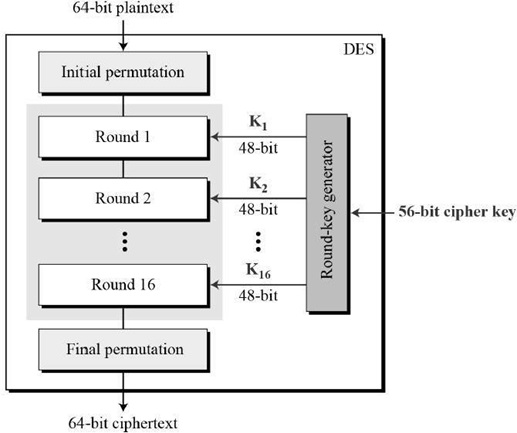Here, DES is depended upon the Feistel Cipher, all that is required to specify DES in that way as,

1. Key Generation
2. Round function
3. Any additional processing − Initial and final permutation

### 1. Key Generation

The round-key generator forms sixteen 48-bit keys out of a 56-bit cipher key in the cryptography. The process of key generation is depicted in the following steps,

• Initially key is of 64 bits.
• Then it goes under PI(1) and after that, it develops key of 56 bits
• Then 56 bit of key divide into two half: left of 28 bit and right of 28 bit.
• In both half do the circular left shift according to the round with we perform.
• As we perform 1,2,9,16 we do circular left shift by one bit and remaining round circular left shift by 2 bit.
• After that combine the left and right half and form again 56 bit of key.
• Then again 56 bit of key goes under PI(2) as form a key of 48 bit.
• This key is used in the DES block diagram.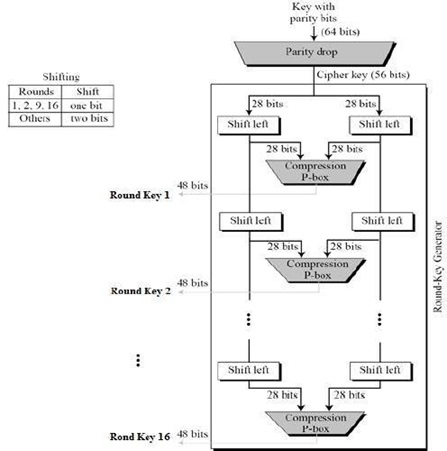### 2. Round Function

The main or important or we can say that the heart of this cipher is the DES function, (f). This DES function applies 48-bit key cryptography to the rightmost 32 bits to produce a 32-bit output as a right.

This function follows some steps,

• Expansion Permutation box
• Xor with the key of 48 bit.
• Substitution Box
• Permutation box.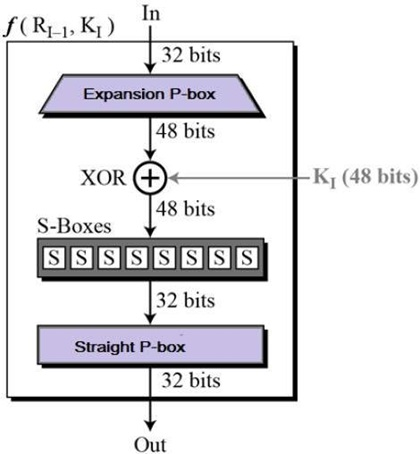• Expansion Permutation Box
Here, right half input is 32-bit and the round key is a 48-bit, we first need to expand right half input to 48 bits.
So, we have to expand the character as we take the 4 bit of 8 characters. In this, we simply convert the 4 bit of each character into 6 bit.
So, we have to expand the 4 bit as in the starting put the bit which presents at the last character of the bit and in the end put the second character first bit.
For example, we have text GIVE ME SOME MONEY, we write it in a four-bit as giving MESO MEMO NEYX now add the bits as tells you further as XGIVEM EMESOM OMEMON ONEYXG and here 16-bit convert into 24 bits.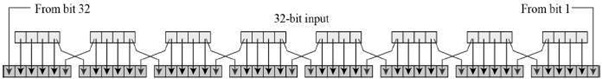• XOR
After that the expansion permutation, DES does XOR operation on the expanded right section of 48 bit and the round key of 48 bit. The round key is used only in this operation and gave the output as 48 bit.
• Substitution Boxes
Thus, the S-boxes carry out the real mixing as confusion. DES uses 8 S-boxes, each with a 6-bit input as plaintext and a 4-bit output as it substitutes it. We have to simply convert 6 bit of character into 4 bit of character.
As we take the first bit or last bit combine as a row and remaining middle four-bit as give a column.
For example, 010010 110010 we take 1 character 00 as 0 rows and 1001 as 9 columns.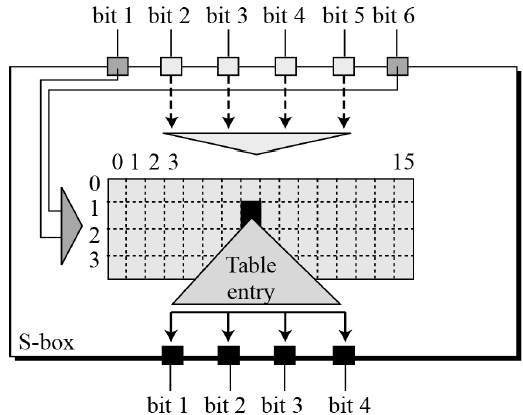• Permutation
The 32-bit output of S-boxes is then subjected to the straight permutation as it has a table according to this we simply update the values by seen in the table and gave an output of 32 bit which further uses as of right.

### 3. Initial and Final Permutation

Simply in starting we take plaintext of 64 bit and divide it into two halves as lest and right each of 32 bits. We use the right half and the key applies to the round function and gave the right half and after then it combines with as Xor of left and right. After that in the second round, left half with came after XOR become right half and original right half become left half and further do this process till round 16.

In the end, the same steps follow and at last left half and right half of 32 bits combine or merge and form a ciphertext of 64 bits in the cryptography in the cryptoanalysis.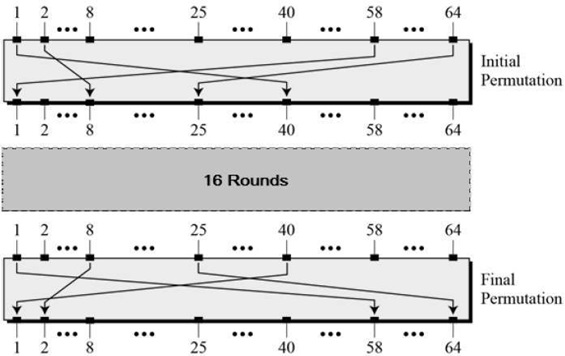## Data Encryption Standard (DES) Decryption Process

It is just the reverse of the encryption process it takes the ciphertext and gave the plain text by just follow the encryption process steps just reverse.

## DES Analysis

This DES satisfies both the desired properties of a block cipher in cryptography. There are two properties make cipher very strong as,

• Avalanche effect: In this, a small change in plaintext results in a very great change in the ciphertext in the cryptography.
• Completeness: In each bit of ciphertext depends on many bits of plaintext in the cryptography.

Image reference(s):

• https://www.tutorialspoint.com/cryptography/data_encryption_standard.htm